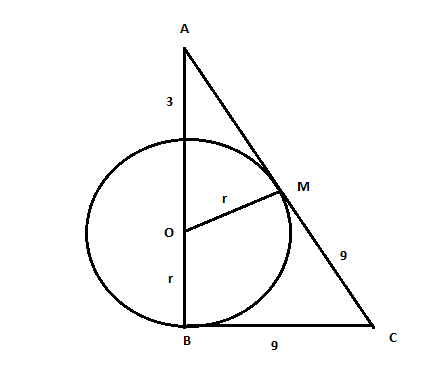Question 11

# A certain city has a circular wall around it, and this wall has four gates pointing north, south, east and west. A house stands outside the city, 3 km north of the north gate, and it can just be seen from a point 9 km east of the south gate. What is the diameter of the wall that surrounds the city?

SolutionB is the south gate. A is the house and BC = 9 km.

In triangle ABC and triangle AMO

ABC = OMA = 90 degree

BAC = OAM = Common angle

Triangle ABC is  similar to triangle AMO.

$$\frac{(2r+3)}{\sqrt{(r+3)^2-r^2}} = \frac{9}{r}$$

r = 9/2

• All Quant CAT Formulas and shortcuts PDF
• 30+ CAT previous papers with solutions PDF